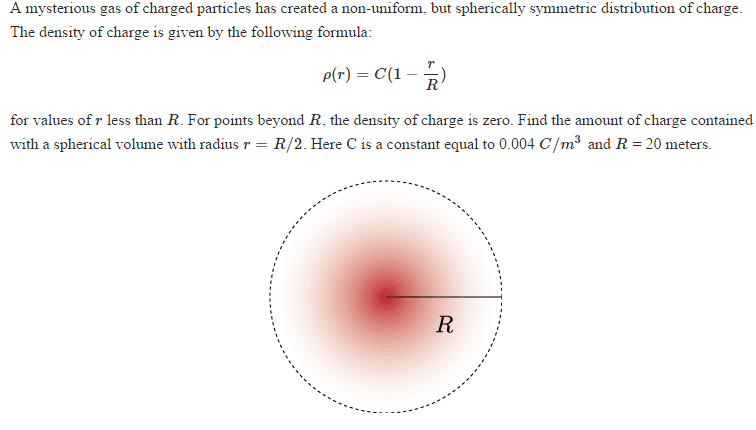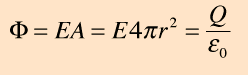# Charge inside a sphere

• goonking
In summary, the electric field at a point 48% of the way from the center of a sphere with charge density of 0.004C/m^3 is 10.47V.f

## Homework Statement## The Attempt at a Solution

so plugging in the variables, the density should be 0.002

with density known, and the volume of the sphere with radius 10, we can find the mass. (density x volume = mass)

it states the amount of charge is symmetric so I assume the number of protons equals to number of electrons.

dividing the mass 16.75 kg by mass of a proton + electron (1.623 x10^-27 kg + 9.11 x 10^-31) = 1.03 x 10^28 pairs of proton and electron

each pair has charge 2q then, so 1.03 x 10^28 x 2(1.602 x10^-19 C) = 3.3 x 10^9 C in the sphere with radius 10.

Is this correct?

Last edited:
By spherically symmetric they mean that it looks the same from any direction. It does not refer to the types of charges involved or their mixture. Given the density function is always positive for any radius, you can assume that the charge is positive everywhere.

There is no mass involved here. The density is in Coulombs per cubic meter. It's a charge density. This density varies with the radial distance from the center, and has the same profile in all directions since it depends only on radius, not direction (hence "spherically symmetric").

You need to work out how much charge (in Coulombs) is contained within a sphere of radius R/2. It's analogous to finding a mass when you integrate the mass density over a volume, but in this case it's charge density you're working with. Note that the density varies with radius: that's the tricky bit.

the density function is always positive for any radius, you can assume that the charge is positive everywhere.

why do we assume this? Can't it have a overall negative charge?

why do we assume this? Can't it have a overall negative charge?
The charge constant in the density formula is ##C = 0.004 C/m^3##. It's a positive constant. The problem doesn't introduce any other details and doing so arbitrarily would only add unnecessary complexity.

so taking out the constants of my integral, i have

(0.002C/m^3) (4π) ∫ r2 dr

my lower and upper bounds are 0 and 10, since the radius of the sphere we are looking at is R/2 = 20/2 = 10

(0.002C/m^3) (4π) r3

(0.002C/m^3) (4π) ((10)3 - (0)3)
I got an answer close to 25.

I don't see the density function in there. Density is not constant throughout the sphere. It varies with the radius according to the given density function.

I don't see the density function in there. Density is not constant throughout the sphere. It varies with the radius according to the given density function.
ok, so it is

C4π ∫(1- (r/20)) (r2) dr

= C4π r( r2 - (r3 /20))

= C4π (r3 - r4/20))

= (.004C/m3) 4π (1000 - 500)

correct?

Check your integration. I think you're forgetting to divide by the exponent value: ##\int x^n dx = \frac{1}{n+1}x^{n+1}##.

Check your integration. I think you're forgetting to divide by the exponent value: ##\int x^n dx = \frac{1}{n+1}x^{n+1}##.

That still looks off to me. Check again.

That still looks off to me. Check again.

r(r2/2 - (r3/3)/20)

= (r3/2 - (r4/3)/20)

unless I did the integral wrong

$\int_{0}^{\frac{R}{2}}4\pi r^2\rho (r)dr\neq 16.755$

or is it

∫(1 -r/20)(r2) dr

= ∫ (r2 - r3/20) dr

= ((r2 - r3/20)^2 ) /2

??

or is it

∫(1 -r/20)(r2) dr

= ∫ (r2 - r3/20) dr

= ((r2 - r3/20)^2 ) /2

??
$\int_{0}^{\frac{R}{2}}4\pi r^2\rho (r)dr=4\pi C\int_{0}^{R/2}r^2\left ( 1-\frac{r}{R} \right )dr=4\pi C\int_{0}^{R/2} r^2-\frac{r^3}{R}dr$
I trust you should be able to do the rest

r(r2/2 - (r3/3)/20)

= (r3/2 - (r4/3)/20)

unless I did the integral wrong
Yeah, I think you need to take another look at what you're integrating:
$$Q = \int_0^{10} 4\pi r^2 C \left(1 - \frac{r}{20} \right) dr$$
Distribute the ##r^2## first, then integrate.

$\int_{0}^{\frac{R}{2}}4\pi r^2\rho (r)dr=4\pi C\int_{0}^{R/2}r^2\left ( 1-\frac{r}{R} \right )dr=4\pi C\int_{0}^{R/2} r^2-\frac{r^3}{R}dr$
I trust you should be able to do the rest
=4πC (103 - 104/80)
= 10.47

Huzzah! Now add the units to your result, perhaps check that the number of significant figures is good, and it'll be done.

Huzzah! Now add the units to your result, perhaps check that the number of significant figures is good, and it'll be done.
Now, considering that same cloud of mysterious charged particles as in the previous problem with the same charge density function, determine the electric field at a point located 48 % of the way to R, (which is still 20 meters). C is also still the same: 0.004 C/m^3

I'm guessing I still need to use an integral for this?

with the bounds 0 to 9.6m (48% of 20 is 9.6)

Now, considering that same cloud of mysterious charged particles as in the previous problem with the same charge density function, determine the electric field at a point located 48 % of the way to R, (which is still 20 meters). C is also still the same: 0.004 C/m^3

I'm guessing I still need to use an integral for this?

with the bounds 0 to 9.6m (48% of 20 is 9.6)

Use Gauss's Law

Use Gauss's LawI'm getting a huge number like 9.24E8

Last edited:
$$\oint E\cdot dS=\frac{\sum Q}{\varepsilon _{0}}$$ Now, its a sphere, so the dot product of E and the normal surface vector is 1, so you can pull E out and the dor product dissapears: $$E\oint dS=\frac{\sum Q}{\varepsilon _{0}}$$ Now $\oint dS$ is simply the surface area of your sphere of radius r. $$\oint dS=4 \pi r^2$$.

Now ask yourself what Q is: its the integral from the last problem, so you must evaluate it at r=.48(20):
$$E\oint dS=\frac{\int_{0}^{.48R}4\pi C\left ( r^2-\frac{r^3}{20} \right )}{\varepsilon _{0}}$$

You should get a huge number, think about it: You got around 10 C for your last answer. C is coulombs, which is an enormous amount of charge. Your answer above isn't correct, its off my an order of magnitude. Try re-doing it the way I mentioned above.